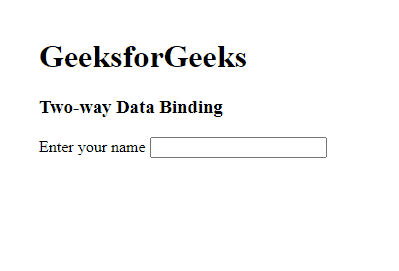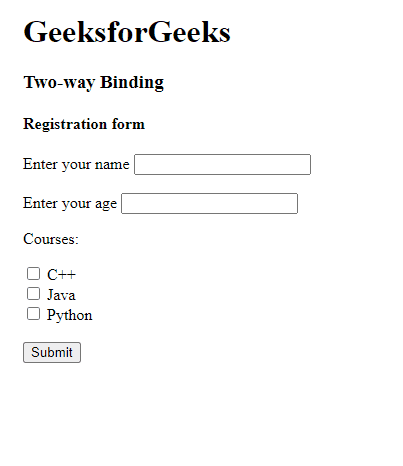GeeksforGeeks App
Open AppBrowser
Continue

## Related Articles

In this article, we will see the Data Binding, along with understanding how the flow of code is from a Typescript file to an HTML file & vice-versa through their implementation.

In AngularJS, Data Binding refers to the synchronization between the model and view. In Two-way data binding, the flow of data is bidirectional i.e. when the data in the model changes, the changes are reflected in the view and when the data in the view changes it is reflected in the model. Two-way data binding is achieved by using the ng-model directive. The ng-model directive transfers data from the view to the model and from the model to the view.

Approach: The following approach will be implemented to achieve the Two-way Binding:

• Create a module
`var app=angular.module('myApp',[])`
• Add a controller to the module. Here you can write the logic for updating the model as the view changes.
`app.controller('myCtrl',function(\$scope){})`
• Specify the ng-model directive in the element
`<input ng-model="name"/>`

Example 1: This example shows how the ng-model directive can be used for two-way data binding.

## HTML

 ```<``html``>`` ` `<``head``>``    ``<``title``>``        ``Two-way Data Binding in AngularJS``    ````    ``<``script` `src``=``"https://cdnjs.cloudflare.com/ajax/libs/angular.js/1.4.7/angular.min.js"``>``    `````` ` `<``body` `ng-app``=``""``>``    ``<``h1``>GeeksforGeeks``    ``<``h3``>Two-way Data Binding``    ``<``form``>``        ``<``label``>Enter your name ``        ``<``input` `type``=``"text"``               ``ng-model``=``"name"``> ``    ````    ``<``p``>{{name}}`````

Explanation: In the above example, we have used the ng-model directive in the input element. When the user enters data in the input element it is reflected in the <p> tag where we have used an expression to display the value of ‘name’. This is an example of two-way data binding as when we change the input control value the value of the variable ‘name’ also changes.

Output:Example 2: In this example, we have created a registration form. The user fills in the details and clicks on the submit button. On clicking on the submit button the details filled by the user are displayed.

## HTML

 ```<``html``>`` ` `<``head``>``    ``<``title``>``        ``Two-way Data Binding in AngularJS``    ````    ``<``script` `src``=``"https://cdnjs.cloudflare.com/ajax/libs/angular.js/1.4.7/angular.min.js"``>``    `````` ` `<``body` `ng-app``=``"myApp"``>``    ``<``h1``>GeeksforGeeks``    ``<``h3``>Two-way Binding``    ``<``h4``>Registration form``    ``<``form` `name``=``"registrationForm"` `          ``ng-controller``=``"myCtrl"``>``        ``<``label``>Enter your name``        ``<``input` `type``=``"text"``               ``ng-model``=``"name"` `/>``        ``<``br` `/>``        ``<``label``>Enter your age``        ``<``input` `type``=``"text"` `               ``ng-model``=``"age"` `/>``        ``<``br` `/>``        ``<``p``>Courses:``        ``<``input` `type``=``"checkbox"``               ``ng-model``=``"value1"` `               ``name``=``"option1"` `               ``value``=``"C++"` `/>``        ``<``label``>C++``        ``<``br` `/>``        ``<``input` `type``=``"checkbox"` `               ``ng-model``=``"value2"` `               ``name``=``"option2"` `               ``value``=``"Java"` `/>``        ``<``label``>Java``        ``<``br` `/>``        ``<``input` `type``=``"checkbox"` `               ``ng-model``=``"value3"` `               ``name``=``"option3"` `               ``value``=``"Python"` `/>``        ``<``label``>Python``        ``<``br` `/>``        ``<``input` `type``=``"submit"` `               ``ng-click``=``"details()"` `/>``        ``<``div``>``            ``<``br` `/>``            ``<``table` `border``=``"1px solid black"` `                   ``style``=``"border-collapse: collapse"``>``                ``<``tr` `border``=``"1px solid black"` `                    ``style``=``"border-collapse: collapse"``                    ``ng-repeat``=``"x in registrationDetails"``>``                    ``<``td``>{{x.key}}``                    ``<``td``>{{x.value}}``                ````            ````        ````    ````    ``<``script` `type``=``"text/javascript"``>``        ``var app = angular.module('myApp', []);``        ``app.controller('myCtrl', function(\$scope) {``            ``\$scope.details = function() {``                ``\$scope.courses = [];``                ``if(\$scope.value1) ``                    ``\$scope.courses.push(registrationForm.option1.value);``                ``if(\$scope.value2) ``                    ``\$scope.courses.push(registrationForm.option2.value);``                ``if(\$scope.value3) ``                    ``\$scope.courses.push(registrationForm.option3.value);``                ``\$scope.registrationDetails = [{``                    ``key: 'Name',``                    ``value: \$scope.name``                ``}, {``                    ``key: 'Age',``                    ``value: \$scope.age``                ``}, {``                    ``key: 'Courses interested in',``                    ``value: \$scope.courses.toString()``                ``}, ];``            ``};``        ``});``    ```````

Output:My Personal Notes arrow_drop_up
Related Tutorials